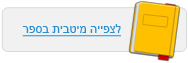עמוד:17 Activity Interactive Simulations Energy Transformations Activity A : Run the Energy of a Bouncing Ball simulation in slow motion ( check the appropriate box ) . Lift the ball , and then release it . Notice how the ball’s velocity changes as the ball rises and falls . The column graph on the left of each illustration depicts the ball’s kinetic energy ( in orange ) , its potential energy ( in blue ) , and their sum . Watch the bouncing ball and the representation of the energy transformations in the column chart . The ball loses potential energy and gains kinetic energy . At this stage , the ball’s potential energy is at its maximum . After hitting the floor , the ball stops and compresses ; its kinetic energy is converted into elastic energy . When the ball hits the floor , it loses all of its potential energy , and its kinetic energy is at its maximum .© מטח - המרכז לטכנולוגיה חינוכית